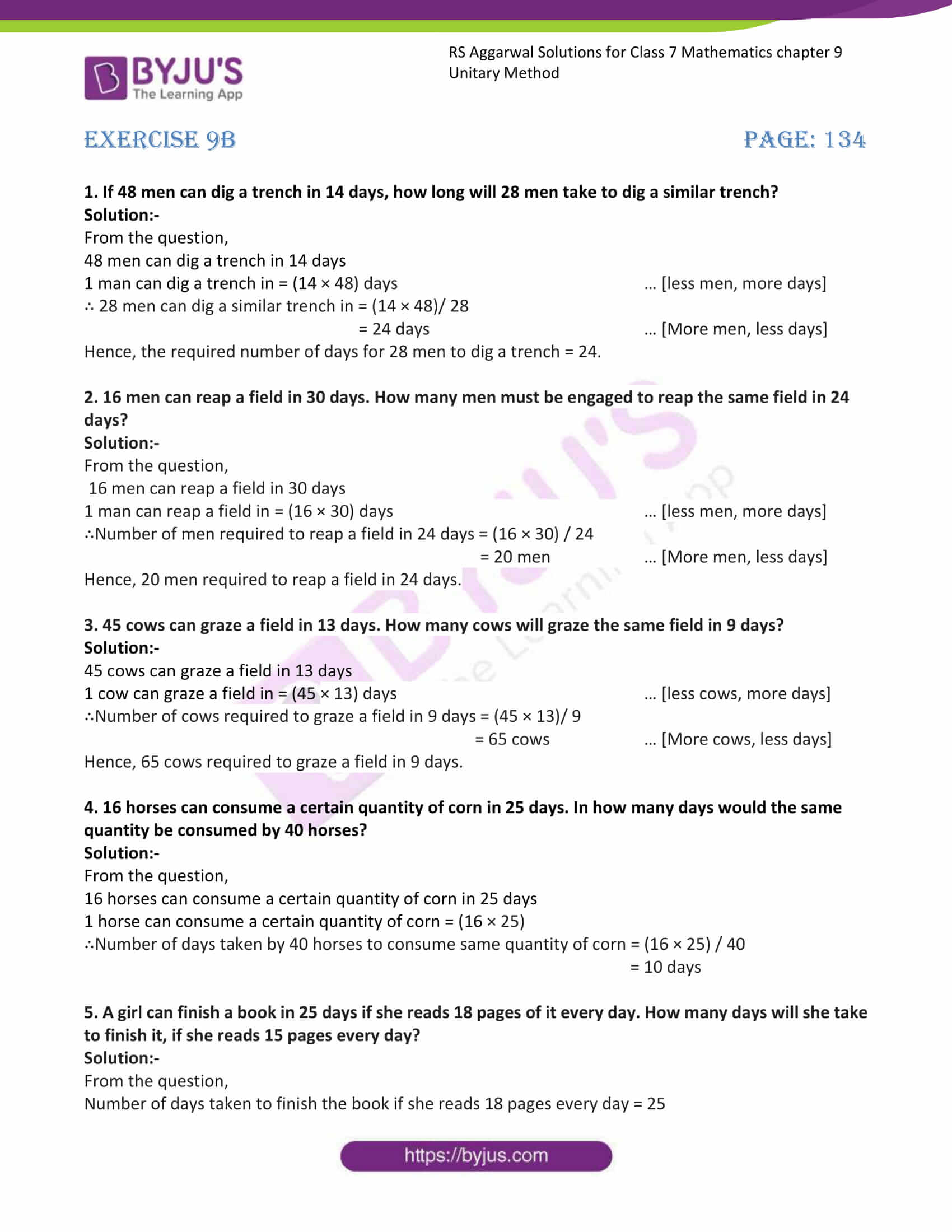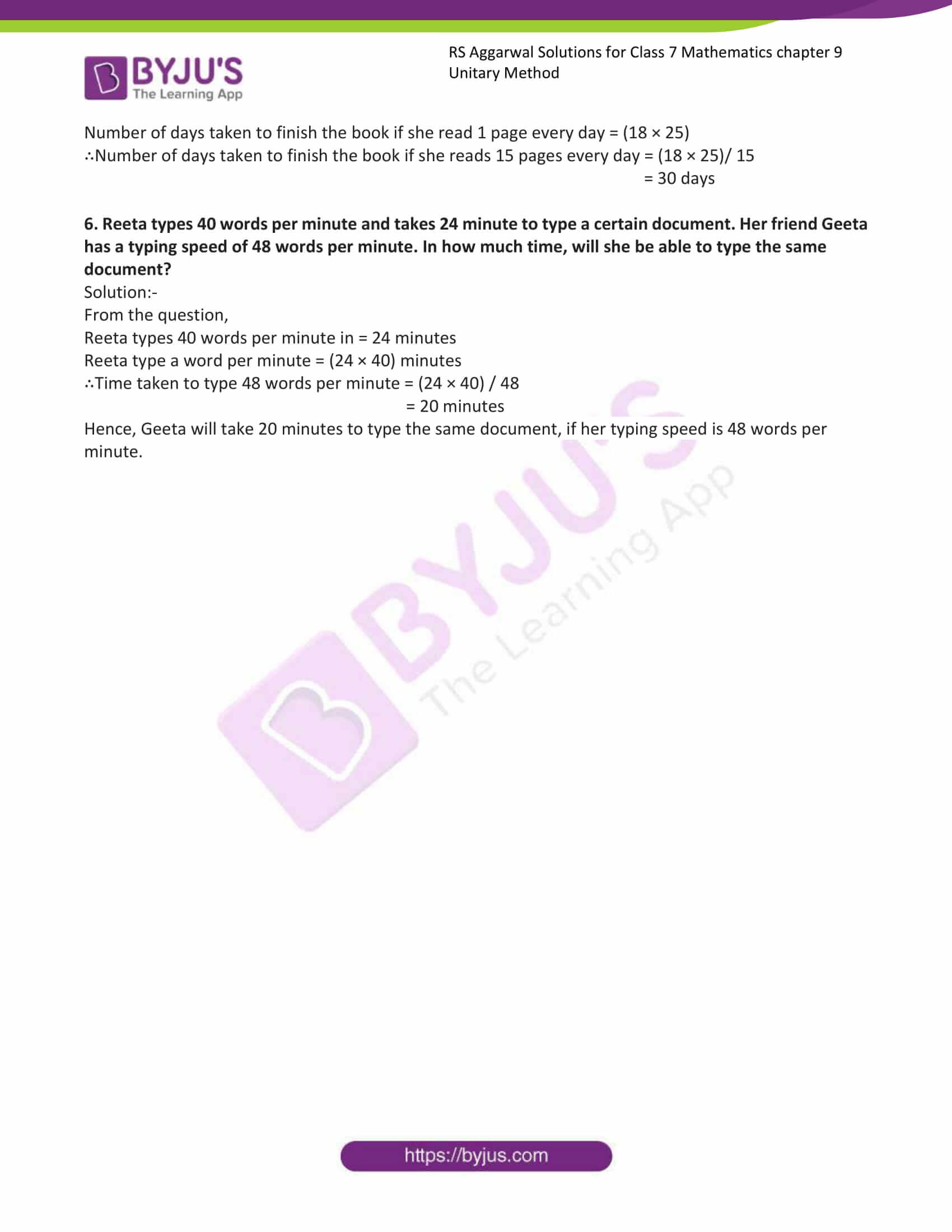# RS Aggarwal Solutions for Class 7 Maths Exercise 9B Chapter 9 Unitary Method

RS Aggarwal Solutions for the Exercise 9B of Class 7 Maths Chapter 9. In this exercise, students are going to solve the problems on Inverse Variation. Two quantities a and b are said to vary inversely if the product ab remains constant. By practising RS Aggarwal Solutions for Class 7 Maths Chapter 9 Unitary Method, students can gain more knowledge about the topic. Students who wish to score good marks in Maths practise RS Aggarwal Class 7 Solutions Chapter 9. Our expert professors formulate these exercises to support you with your exam preparation and come out with flying colours.

## Download the PDF of RS Aggarwal Solutions For Class 7 Maths Chapter 9 Unitary Method – Exercise 9B### Access answers to Maths RS Aggarwal Solutions for Class 7 Chapter 9 – Unitary Method Exercise 9B

1. If 48 men can dig a trench in 14 days, how long will 28 men take to dig a similar trench?

Solution:-

From the question,

48 men can dig a trench in 14 days

1 man can dig a trench in = (14 × 48) days … [less men, more days]

∴ 28 men can dig a similar trench in = (14 × 48)/ 28

= 24 days … [More men, less days]

Hence, the required number of days for 28 men to dig a trench = 24.

2. 16 men can reap a field in 30 days. How many men must be engaged to reap the same field in 24 days?

Solution:-

From the question,

16 men can reap a field in 30 days

1 man can reap a field in = (16 × 30) days … [less men, more days]

∴Number of men required to reap a field in 24 days = (16 × 30) / 24

= 20 men … [More men, less days]

Hence, 20 men required to reap a field in 24 days.

3. 45 cows can graze a field in 13 days. How many cows will graze the same field in 9 days?

Solution:-

45 cows can graze a field in 13 days

1 cow can graze a field in = (45 × 13) days … [less cows, more days]

∴Number of cows required to graze a field in 9 days = (45 × 13)/ 9

= 65 cows … [More cows, less days]

Hence, 65 cows required to graze a field in 9 days.

4. 16 horses can consume a certain quantity of corn in 25 days. In how many days would the same quantity be consumed by 40 horses?

Solution:-

From the question,

16 horses can consume a certain quantity of corn in 25 days

1 horse can consume a certain quantity of corn = (16 × 25)

∴Number of days taken by 40 horses to consume same quantity of corn = (16 × 25) / 40

= 10 days

5. A girl can finish a book in 25 days if she reads 18 pages of it every day. How many days will she take

to finish it, if she reads 15 pages every day?

Solution:-

From the question,

Number of days taken to finish the book if she reads 18 pages every day = 25

Number of days taken to finish the book if she read 1 page every day = (18 × 25)

∴Number of days taken to finish the book if she reads 15 pages every day = (18 × 25)/ 15

= 30 days

6. Reeta types 40 words per minute and takes 24 minute to type a certain document. Her friend Geeta

has a typing speed of 48 words per minute. In how much time, will she be able to type the same

document?

Solution:-

From the question,

Reeta types 40 words per minute in = 24 minutes

Reeta type a word per minute = (24 × 40) minutes

∴Time taken to type 48 words per minute = (24 × 40) / 48

= 20 minutes

Hence, Geeta will take 20 minutes to type the same document, if her typing speed is 48 words per

minute.

### Access other exercises of RS Aggarwal Solutions For Class 7 Chapter 9 – Unitary Method

Exercise 9C Solutions